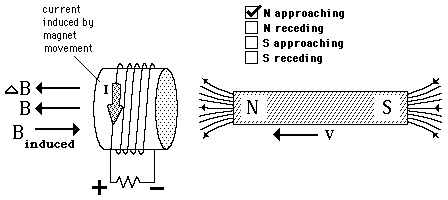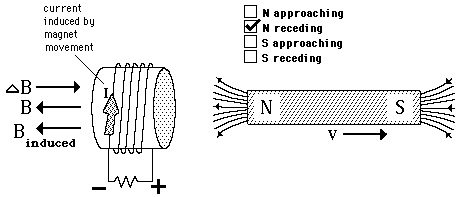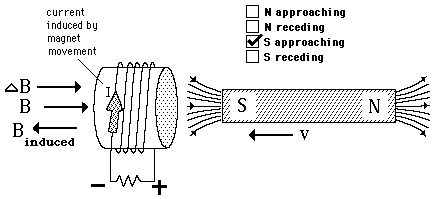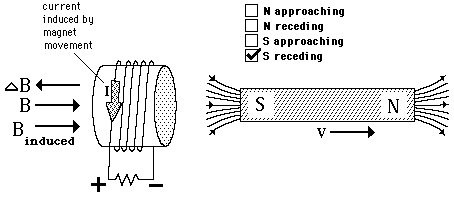# Generated Voltage from Changing BThe induced emf (generated voltage) can be calculated from Faraday's Law. According to Lenz's Law the induced current in the coil produces a magnetic field to oppose the change in magnetic field which produced it. Observing the direction of the change in the magnetic field of the bar magnet, you know that the induced current will produce a magnetic field in the opposite direction called the induced field. If you put the fingers of your right hand inside the coil in the direction of that induced field, the thumb points in the direction the current must flow to produce the field.

 Field of current loop
Index

Magnetic field concepts

Currents as magnetic sources
HyperPhysics***** Electricity and Magnetism Go Back

# Generated Voltage from Changing BThe induced emf (generated voltage) can be calculated from Faraday's Law. According to Lenz's Law the induced current in the coil produces a magnetic field to oppose the change in magnetic field which produced it. Observing the direction of the change in the magnetic field of the bar magnet, you know that the induced current will produce a magnetic field in the opposite direction called the induced field. If you put the fingers of your right hand inside the coil in the direction of that induced field, the thumb points in the direction the current must flow to produce the field.

 Field of current loop
Index

Magnetic field concepts

Currents as magnetic sources
HyperPhysics***** Electricity and Magnetism Go Back

# Generated Voltage from Changing BThe induced emf (generated voltage) can be calculated from Faraday's Law. According to Lenz's Law the induced current in the coil produces a magnetic field to oppose the change in magnetic field which produced it. Observing the direction of the change in the magnetic field of the bar magnet, you know that the induced current will produce a magnetic field in the opposite direction called the induced field. If you put the fingers of your right hand inside the coil in the direction of that induced field, the thumb points in the direction the current must flow to produce the field.

 Field of current loop
Index

Magnetic field concepts

Currents as magnetic sources
HyperPhysics***** Electricity and Magnetism Go Back

# Generated Voltage from Changing BThe induced emf (generated voltage) can be calculated from Faraday's Law. According to Lenz's Law the induced current in the coil produces a magnetic field to oppose the change in magnetic field which produced it. Observing the direction of the change in the magnetic field of the bar magnet, you know that the induced current will produce a magnetic field in the opposite direction called the induced field. If you put the fingers of your right hand inside the coil in the direction of that induced field, the thumb points in the direction the current must flow to produce the field.

 Field of current loop
Index

Magnetic field concepts

Currents as magnetic sources
HyperPhysics***** Electricity and Magnetism Go Back